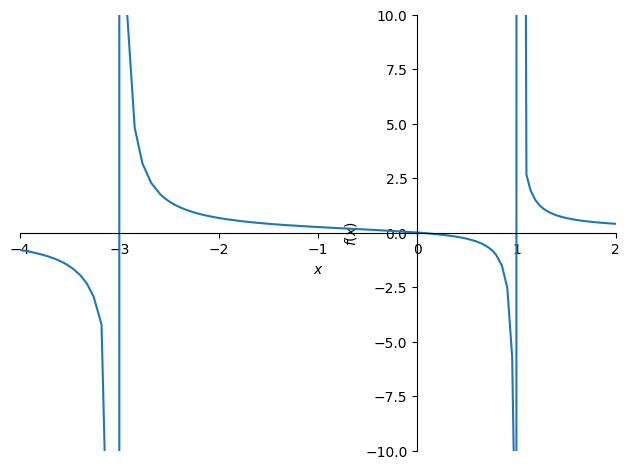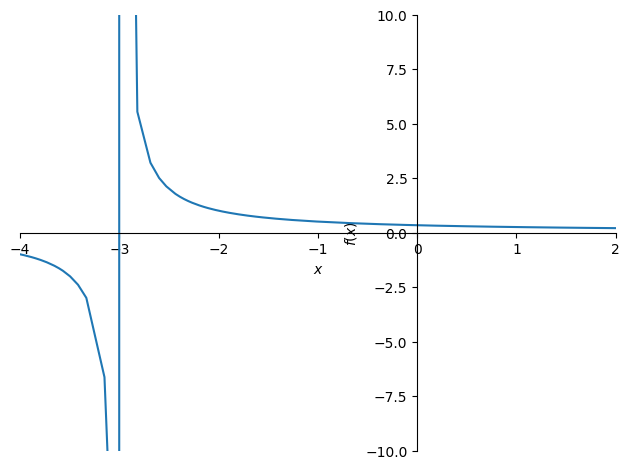:

from sympy import init_session
init_session(quiet=True)




# Limits

## Syllabus Goals

Working on this through week ending October 9, 2022.

“The problems are the solution.” (Johnsin University B.S. Program Guide)

Assignments:

• Paul’s Math Notes on L’Hospital’s Rule.

• Review Asympotes vs Holes Professor Leonard Notes. Organize some sample problems around this.

Try for example on

$\frac{\sin{x}}{x}$

or other hard-to-do problems. Inductively work with L’Hospital’s rule.

## Different Types of Limits:

• Non-rational polynomials are easy, can always do by substitution. e.g. $$\lim_{x\to2} 3x^2 + 2x - 9 = 7$$. Paul’s math notes expresses this formally thus: If p(a) is a polynomial, then $$\lim_{x\to a} p(x) = p(a)$$.

### For rational polynomials:

• If substituting gives non-zero in numerator and denominator, easy peasy, $$\lim_{x\to2}\frac{3x^2 + 2x - 9 = 24}{2x +3} = \frac{7}{7} = 1$$

• Zero in numerator only is fine. So for example $$\lim_{x\to2}\frac{x^2 - 4}{3x + 7} = \frac{0}{7} = 0$$ Note also in Python, print(0/anything) = 0.0.

• Zero in denominator only is an asymptote or DNE because asymptotes are one-sided. That is, answer will be always be either $$\infty$$, $$- \infty$$, or $$\pm\infty$$ depending on the direction, right-hand limit, left-hand limit, etc. The sign is what tells you what’s happening at each direction. The proof is, if you’re approaching zero, you’re dividing by, for example $$\frac{1}{10},\frac{1}{100},...$$ Dividing by fraction = multiplying by reciprocal of denominator, ie. by $$10, 100, 1000, 10000...$$. So you’re going toward infinity. For example $$\lim_{x\to2}\frac{3x + 7}{x^2 - 4} = \:?$$. See the graph. Because of this, you have to check denominator by plugging in values > and < x (informal “sign analysis”). Any easy values above and below are OK. For example, given denom of $$x^2 - 4$$, if x is 1, get $$1^2 - 4 = 1 - 4 = -3$$, negative. But for x = 3, get $$3^2 - 4 = 9 - 4 = 5$$, positive. And sure enough, graph shows $:nbsphinx-math:lim_{x:nbsphinx-math:to0^{+}} = +:nbsphinx-math:infty$ and :math:lim_{xto0^{-}}= -infty but the limit does not exist.

(See limit notes below on functions at infinity – relearned today in Goodman’s Barron’s Calculus section 3.3.)

Continuity and Limits from Paul’s Math Notes: ## Definition of continuity A function $$f(x)$$ is continuous at $$x = a$$ if

$\lim_{x\to a} f(x) = f(a)$

A function is said to be continuous on the interval $$[a,b]$$ if it is continuous at each point on the interval.

The corrolary of this is that if $$f(x)$$ is continuous at $$x = a$$, then the following is true:

$\lim_{x\to a} f(x) = \lim_{x\to a+} f(x) = \lim_{x\to a-} f(x) = f(a)$
:

# Checking math on above in SymPy
from sympy.plotting import plot
print(limit(3*x**2 + 2*x - 9, x, 2))
print(limit((3*x**2 + 2*x - 9)/(2*x + 3), x, 2))
print(limit((x**2 - 4)/(3*x + 7), x, 2))

7
1
0


## Weekly review

• Sat did about 1 hour on above notes.

• Sun 1.5 hours

• Mon 3 hours. Hard, and not much writing done.

• Tues 2.5 Hours.

• Wed 2.0 hours 1.5 reviewing limits and a Set Theory Youtube introduction

• Thur 2-ish (began working again on Derivatives)

• Fri TBD

12 hours (Not counting Friday – TBD)

Getting to three hours per day didn’t really work, and cut a whole in writing (see Monday). Mostly the limits work went well, lots of problems done early in the week. Slowed down on quadratic review. Did not really do much with L’Hopital yet.

## Limits Notes (Original)

This part of notes from Calc 1 3.5 Limits of Functions at Infinity. When numerator equals zero, that’s a point, zero, fine. When denominator equals zero, that’s a discontinuity.

It’s a removeable discontinuity if you can factor it; you can tell this often if you get $$\frac{0}{0}$$. Otherwise it’s an asymptote – can’t be canceled, only in denom.

We should be able to show this in Sympy.

$y = mx +b$
:

f = x / ( (x + 3) * (x - 1) )
f

:

$\displaystyle \frac{x}{\left(x - 1\right) \left(x + 3\right)}$

This should have two asymptotes at + 1 and -3 (NOT at zero, numerator is fine).

:

%matplotlib inline
plot(f, xlim=(-4, 2), ylim=(-10, 10)):

<sympy.plotting.plot.Plot at 0x10fb0ded0>


For the following function, the x-1 gets cancelled out. So this has ONE asymptote at -3.s

:

%matplotlib inline
g = (x - 1) / ((x + 3) * (x - 1) )
g

:

$\displaystyle \frac{1}{x + 3}$
:

plot(g, xlim=(-4, 2), ylim=(-10, 10)):

<sympy.plotting.plot.Plot at 0x10f1b0d90>


To determine which way it’s going, just plug in numbers along interval. So given asymptotes at -3, 1, can plug in values for intervals > 1 and < -3.

:

[f.subs(x, i) for i  in (-4, -.5, .5, 2)]

:

$\displaystyle \left[ - \frac{4}{5}, \ 0.133333333333333, \ -0.285714285714286, \ \frac{2}{5}\right]$
:

f.subs(x, -4)

:

$\displaystyle - \frac{4}{5}$
:

So limit doesn't exist at -3 or 1, because not going in same direction.  Limit DOES exist at zero, because it's a point.

  Cell In, line 1
So limit doesn't exist at -3 or 1, because not going in same direction.  Limit DOES exist at zero, because it's a point.
^
SyntaxError: invalid syntax



## Example

[ ]:

h = t**3 / (t**2 -1)**2
h


By factoring t squared - 1, get:

[ ]:

(t + 1)*(t- 1)


So discontinuities at 1, -1. these are asymptotes. So we could substitute:

[ ]:

[h.subs(t, i) for i  in (-2, -.5, .5, 2)]


So my guess is we’ll get limits at negative infinity, infinity. I was right about guess I think but graph looks like hell in this case. Looks better on Desmos

[ ]:

%matplotlib inline
plot(h, xlim=(-3, 3), ylim=(-100, +100), )


Next he asks what happens as x –> + oo or x –> - oo, for example:

$\lim_{x \to -\infty} \frac{1}{x}$
$\lim_{x \to \infty} \frac{1}{x}$

Using a table x, f(x) for 1, 10, 100, 10000000, showed that limit approaching positive infinity is zero. Same for negative infinity case.

So if f(x) gets “really close” to a certain number as x -> +/- oo, then the LIMIT EXISTS. But what does it mean? So you end up with a HORIZONTAL asymptote.

So:

• Vertical asymptote: When you have a non-removal discontinuity.

• Horizontal asymptote: if you have a limit for f(x) as x gets really close to a certain number as x -> +/- infinity.

Also, because we can pull out any exponent, any constant / some variable to the nth power e.g.

$\frac{1}{x^n}$

The limit of polynomial as x -> +/- oo:

Polynomials always have tails going up or down or both. Never actually go to a number, they go to infinity in one direction or other as x goes to +/- infinity.

[ ]:

# Scratch
cos(0)
cos(.00001)

[ ]:

cos(.001)

[ ]:

cos(-.001)

[ ]: